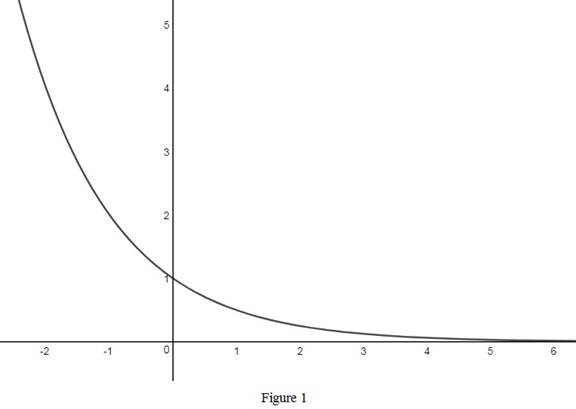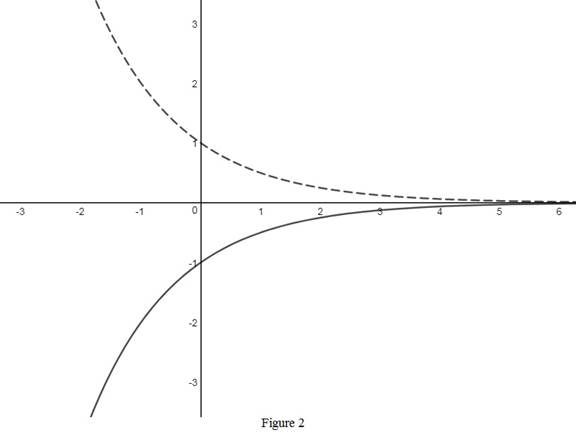# The rough graph of the function y = − 2 − x use transformations if needed.### Single Variable Calculus: Concepts...

4th Edition
James Stewart
Publisher: Cengage Learning
ISBN: 9781337687805### Single Variable Calculus: Concepts...

4th Edition
James Stewart
Publisher: Cengage Learning
ISBN: 9781337687805

#### Solutions

Chapter 1.5, Problem 13E
To determine

## To sketch: The rough graph of the function y=−2−x use transformations if needed.

Expert Solution

### Explanation of Solution

Rewrite the given function as y=(12)x .

The standard graph of the function y=(12)x is roughly sketched as shown below in Figure 1.Then, to draw the graph of y=(12)x , reflect the graph y=(12)x about thex-axis. Thus, the graph of y=(12)x is shown below in Figure 2.Observe that Figure 2 is obtained from Figure 1 in such a way that it is reflected about the x-axis.

### Have a homework question?

Subscribe to bartleby learn! Ask subject matter experts 30 homework questions each month. Plus, you’ll have access to millions of step-by-step textbook answers!